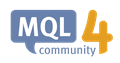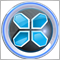# Custom Fibonacci Problem636

HI guys,

I can display the default fib on charts no problem but if I want a custom set of levels I run into problems. I read the documentation here https://docs.mql4.com/constants/objectconstants/enum_object/obj_fibo which shows the code but doesn't actually use it in the example!

Can anybody offer any ideas as to where I have gone wrong please?

```void OnStart()
{
datetime time1, time2;
double price1, price2;
int chart_ID = 0;
string name = "fibname";
double fib_values = {0.0,23.6,38.2,50.0,55.0,61.8,78.6,100.0,127.2,141.1,161.8,261.8,423.6};

time1 = iTime(NULL,0,1);
time2 = time1;
price1 = iLow(NULL,0,1);
price2 = iHigh(NULL,0,
1);

//--- Create Fibonacci Retracement by the given coordinates
if(!ObjectCreate(chart_ID,name,OBJ_FIBO,0,time1,price1,time2,price2))
{
Print(__FUNCTION__,
": failed to create \"Fibonacci Retracement\"! Error code = ",GetLastError());
return;
}
ObjectSetInteger(chart_ID,name,OBJPROP_FIBOLEVELS,13);

for(int i=0;i<13;i++)
{
Print(fib_values[i]);
//--- level value
ObjectSetDouble(chart_ID,name,OBJPROP_FIRSTLEVEL+i,fib_values[i]);
}
ObjectSetInteger(chart_ID,name,OBJPROP_COLOR,Magenta)
ObjectSetInteger(chart_ID,name,OBJPROP_STYLE,STYLE_SOLID);
ObjectSetInteger(chart_ID,name,OBJPROP_WIDTH,1);
ObjectSetInteger(chart_ID,name,OBJPROP_RAY_RIGHT,False);

return;

}```OBJ_FIBO - Object Types - Objects Constants - Constants, Enumerations and Structures - MQL4 Reference
• docs.mql4.com
The following script creates and moves Fibonacci Retracement on the chart. Special functions have been developed to create and change graphical object's properties. You can use these functions "as is" in your own applications. //| Create Fibonacci Retracement by the given coordinates            |               time1=0,           ...29403

So this section should not be same date time:

```time1 = iTime(NULL,0,1);
time2 = time1;```

it can be like :

```time1 = iTime(NULL,0,1);
time2 = iTime(NULL,0,10);```
Do as documentation example carefully.636

So this section should not be same date time:

it can be like :

Do as documentation example carefully.

So how do you put a fibonacci on a single candle? It has the same time for points 1 & 2?

Different price 1 & 2.29403

OK,So main issue is the levels,you should divide your levels by 100,then spend it to fibo value array ,like this:

```void OnStart()
{
datetime time1,time2;
double price1,price2;
int chart_ID= 0;
string name = "fibname";
double fib_values={0.0,.236,.382,.500,.550,.618,.786,1.000,1.272,1.411,1.618,2.618,4.236};

time1 = iTime(NULL,0,1);
time2 = iTime(NULL,0,2);
price1 = iLow(NULL,0,1);
price2 = iHigh(NULL,0,1);

//--- Create Fibonacci Retracement by the given coordinates
if(!ObjectCreate(chart_ID,name,OBJ_FIBO,0,time1,price1,time2,price2))
{
Print(__FUNCTION__,
": failed to create \"Fibonacci Retracement\"! Error code = ",GetLastError());
return;
}
ObjectSetInteger(chart_ID,name,OBJPROP_FIBOLEVELS,13);

for(int i=0;i<13;i++)
{
Print(fib_values[i]);
//--- level value
ObjectSetDouble(chart_ID,name,OBJPROP_FIRSTLEVEL+i,fib_values[i]);
ObjectSetString(chart_ID,name,OBJPROP_LEVELTEXT,i,DoubleToString(100*fib_values[i],1));
}
ObjectSetInteger(chart_ID,name,OBJPROP_COLOR,Magenta);
ObjectSetInteger(chart_ID,name,OBJPROP_STYLE,STYLE_SOLID);
ObjectSetInteger(chart_ID,name,OBJPROP_WIDTH,1);
ObjectSetInteger(chart_ID,name,OBJPROP_RAY_RIGHT,False);

return;

}```

If you are using it in an indicator the main event handler function should be OnCalculate() and also for EA should be OnTick(),

Regards.636

OK,So main issue is the levels,you should divide your levels by 100,then spend it to fibo value array ,like this:

If you are using it in an indicator the main event handler function should be OnCalculate() and also for EA should be OnTick(),

Regards.

Thank you very much.29403

sd59:

Thank you very much.

You're welcome.